# Invention of Napier’s logarithm

• I
• DLeuPel
In summary, logarithms were invented by John Napier through the use of comparing arithmetic and geometrical progressions. The concept of a point traveling along a segment with decreasing velocity led to the idea of logarithms. The formula for finding logarithms was later developed using initial conditions and the concept of proportionality. This laid the foundation for the invention of the slide rule, which made multiplication easier. The concept of logarithms has also had a significant impact in mathematics and other fields.

#### DLeuPel

I’m trying to figure out how logarithms we’re invented. In addition, what does the calculator do when I want to solve a logarithm. After researching I found out that you could compare an arithmetic progression with a geometrical one, obtaining the principal properties of exponent calculation. Later, I found that Napier imagined a segment AB, where there was a point Q traveling along the segment but it’s velocity decreased proportionally in relation to the distance left to reach B. This would be a geometric progression. Then, you would divide the segment for each second that passed. After that, you would stretch the divided parts so they are equal. So due to the fact that the velocity decreases in relation to the distance left to B, it never reaches B. Therefore, the distance to B is infinite since for each second, the point travels the same distance. This would be an arithmetical progression.

Until there I can sort of understand it, but the difficult part for me comes here:

—————- The point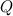moves at a constant speed of 107, so we have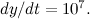Since P moves at a speed that is proportional to the distance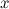left to travel we have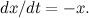From this we see that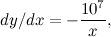which gives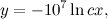for some constant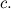We can work out the value of c using our initial conditions. At the start, the point P still needs to travel the whole length of the line segment AB, which is 107. Therefore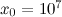. The point Q hasn’t gone anywhere yet, so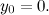Plugging this into the expression above gives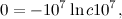so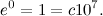Therefore,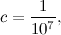so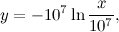and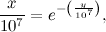————-

All of the calculations on the top are copied from https://plus.maths.org/content/calculating-napiers-logarithm

Now, if we are trying to solve or to figure out how were logarithms invented and imagined, how does the ln appear if that itself is a logarithm. Also, could someone complete the explication of the foundation of logarithms ?

#### Attachments

DLeuPel said:
Now, if we are trying to solve or to figure out how were logarithms invented and imagined, how does the ln appear if that itself is a logarithm. Also, could someone complete the explication of the foundation of logarithms ?
Well, multiplication used to be hard and addition easy (by comparison). Therefore, the invention of logarithms meant that you could look up the factors in a table, add them, and then look up the answer in (another) table.

And - it laid the groundwork for the invention of the "slide rule", the essential part of any engineer's tool set.

Svein said:
Well, multiplication used to be hard and addition easy (by comparison). Therefore, the invention of logarithms meant that you could look up the factors in a table, add them, and then look up the answer in (another) table.

And - it laid the groundwork for the invention of the "slide rule", the essential part of any engineer's tool set.
I was thinking more of a mathematical approach for an explanation, but thank you.

•pbuk